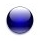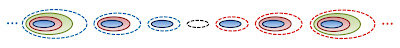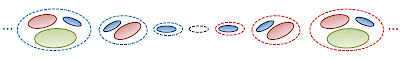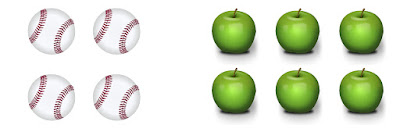This blog has moved ! New look and new articles on www.naturelovesmath.com/en/

# About numbers, episode 2 : Integers

 Easy : for the curiousNegative numbers (Source Wikipedia commons)
A quantity of -2 objects, a marathon runner finishing -3rd, numbers smaller than nothing ?! What do negative numbers mean ?

The answer to that question is easy only to those who are used to them. And for most of us, it doesn't matter what they really are or what they mean, because the thing is these numbers are quite useful !

However, for the ancient Greeks as well as for the Arab and Indian mathematicians - who already understood the meaning of the zero and the negative numbers -, the negative roots of an equation are thought of being unnatural, absurd even according to Nicolas Chuquet (~1450-1488) and would not be recognized until the XIXth and XXth century ! So useful, but also very problematic...

We will see that their meaning is more subtle than we would think, and that it is illuminating to base it on the distinction between ordinals and cardinals that we made in the preceding chapter about natural numbers

The ordinal... integers

A first approach of integers can lean on the concept of natural ordinal number. We saw that a natural ordinal really is just an ordered set. A well-ordered set to be precise. We say that a set is well-ordered if every subset has a least element for that order. Indeed, every subset of natural numbers has a least element, and the set of all natural numbers has zero as least element.
In a way, we order an infinite set of elements "in one direction" from one privileged element.
Let's construct the integers by ordering "in two directions" from one privileged element.

We recall the principles of construction of ordinal natural numbers, using set theory's concepts.
First, let's call "ordinal number 0" the empty set noted $\color{red}{\varnothing}$.
The next ordinal number, 1, is the powerset of $\color{red}{\varnothing}$, that is to say the set $\color{red}{\{\varnothing\}}$ containing only $\color{red}{\varnothing}$.

The next ordinal number, 2, is the powerset of $\color{red}{\{\varnothing\}}$, that is to say the set $\color{red}{\{\varnothing,\{\varnothing\}\}}$. It contains "0" the empty set $\color{red}{\varnothing}$ and "1" its powerset $\color{red}{\{\varnothing\}}$.

And we continue, the next ordinal, 3, is $\color{red}{\{\varnothing,\{\varnothing\},\{\varnothing,\{\varnothing\}\}\}}$.

Etc.

Why the red colored brackets and numbers ? Well, because we are going to do the same thing one more time, and the color will help us distinguish them. Let's do it again with, say, the color blue :

This time, we are going to define the ordinal number preceding 0.
So 1, is the powerset of $\color{blue}{\varnothing}$, that is to say the set $\color{blue}{\{\varnothing\}}$ containing only $\color{blue}{\varnothing}$.

The preceding ordinal number, 2, is the powerset of $\color{blue}{\{\varnothing\}}$, that is to say the set $\color{blue}{\{\varnothing,\{\varnothing\}\}}$. It contains "0" the empty set $\color{blue}{\varnothing}$ and "1" its powerset $\color{blue}{\{\varnothing\}}$.

And we continue, the next ordinal, 3, is $\color{blue}{\{\varnothing,\{\varnothing\},\{\varnothing,\{\varnothing\}\}\}}$.

Etc.Construction of ordinal integers

We just constructed in red the successors of zero and in blue its predecessors. The last thing to do is to formally define the order of our colored numbers, because any property that seems "obvious" could potentially backfire. A blue number is always smaller than a red one, and two red numbers are ordered "from smallest to largest", while the blue ones are ordered "from largest to smallest" 1. It's all good, we have a well-defined total order, but note that there is no least colored number for this order...

The blue numbers are of course negative numbers. But what do they mean ?
Instead of the color difference, we could have used other symbols as well, like roman numerals. We then would have the following sequence

... VI, V, IV, III, II, I, 0, 1, 2, 3, 4, 5, 6 ...

Let's push our imagination further. We could use any symbols to represent them, for exemple a mixture of numerals in several languages :Numbers from around the world 2

Our reference point (zero) is the "football ball" at the center (it is the zero Maya). We could even use symbols with no link to numeration whatsoever, or any object for that matter, preceding and following a central object... The point is that the order and the central element are what's important here, and not the nature of the symbols or the objects they refer to.

In a way we are just ordering an infinity of objects "in two directions" from a particular element.

That's why it doesn't make sense to talk about a marathon runner who finished -3rd ! Because we only order the runners "in one direction" !Order of a marathon

The fact that the zero is not attributed to any runner is just a choice, it wouldn't pose any problem to use it. Only the podiums would look different :Weird podium, but the order's the same ! Source : Svilen milev via Wikipedia commons

Now let's try to order the runners of a marathon "in two directions". It means that we have to order them from a particular runner, and not from the finish line.Relative order of a marathon

Such a "relative" order doesn't seem very useful, because the order of a marathon is interesting only if there is a least element, a winner... Unless maybe the marathon is based on a system of points relative to a particular runner for example.
It is exactly the same thing when we order (without counting them) the orbits of the earth around the sun, and we see then all the use of integers : since it is impossible for us to order these orbits from the first one (when was the first one ? since the earth formed progressively, there is no answer to this question), we have then chosen a particular event as a reference point (the zero), and we settled to order the years from it... Or, kinda settled. There exists calendars based on different events (Egyptian, Persian, Roman, Gregorian, etc.), or even some that are not based on the earth orbits around the sun (Muslim lunar calendar, Hebraic or Chinese lunisolar calendar, etc.). But all of them are relative to a particular event of reference. Here are three examples 3 :Three calendars

The cardinal... integers

So we can imagine a setting in which it makes sense for a runner to finish -3rd, like in the course of the years, but what about counting negative quantities ? Well, it's a bit more subtle.
Beforehand, let's try to generalize the concept of cardinal number, the same way we generalized that of ordinal number.
Remember we define cardinal numbers using ordinal ones :

The cardinal number 0 is the number of elements of the set $\color{red}{\varnothing}$ the ordinal 0.
The cardinal number 1 is the number of elements of the set $\color{red}{\{\varnothing\}}$ the ordinal 1.
The cardinal number 2 is the number of elements of the set $\color{red}{\{\varnothing,\{\varnothing\}\}}$ the ordinal 2.
etc.

Let's do it again in blue :
The cardinal number 0 is the number of elements of the set $\color{blue}{\varnothing}$ the ordinal 0.
The cardinal number 1 is the number of elements of the set $\color{blue}{\{\varnothing\}}$ the ordinal 1.
The cardinal number 2 is the number of elements of the set $\color{blue}{\{\varnothing,\{\varnothing\}\}}$ the ordinal 2.
etc.Construction of cardinal integers

So we count not just one, but two distinct quantities. But these can be completely different, with no link whatsoever, for example the number of marathon runners who have finished the run at a given instant (red numbers), and the number of coins in your pocket right now as you read these lines (blue number)... Which, I agree with you, is not very interesting. But we will see that we can give a meaning to the arithmetics on these two unrelated quantities, by means of comparison.

Saying one has got -2 apples doesn't mean a thing if one only counts the number of apples. For that negative quantity to have a meaning, one must count the apples at the same time as, let's say, staples. So to say one has got -2 apples can mean that one has two staples more than he's got apples... Here we make a comparison, a relative counting.

It's however more interesting to count two linked quantities, even directly "opposite" ones like the number of runner who passed the finish line at a given instant (red numbers) and the number of those who aren't finished yet (blue numbers), independently of order (that's what differentiates them from ordinal numbers). In particular, what will interest us is to compare the number of finished runners relatively to those still running. We will get back to this when we see the arithmetics of cardinals. Let's first see that of ordinals.

The arithmetics of ordinal integers

We saw in the article about natural numbers that addition is the fittest operation for these numbers. To add an ordinal number is to "progress" in the ordering. This is of course still true for integers, but we can now define a new operation : subtraction.
To subtract an ordinal is to "regress" in the ordering. Indeed, for every order (for example $\leq$) there exits an inverse order (with our example that would be $\geq$) and to subtract is to "progress for the inverse order" ! So it is addition's inverse operation.Two operations : addition and subtraction

Since these operations are each other's inverse, to subtract and then add a same number (and conversely) sets back to zero. Take two steps forward and then two backward does not take us very far, does it ? With our colored notation, 1+1=0. Also 2+2=0 and generally x+x=0 for every natural number x.
But don't let the notation fool you ! Remember that blue numbers are the negatives we know well (too well according to my banker), so we haven't written anything absurd, just that 1-1=0, 2-2=0, etc.

In the notation of set theory, it means that identical blue sets and red sets cancel each others out. Like matter and antimatter, when put together they annihilate each other !
For example, $\{\color{red}{\varnothing},\color{blue}{\varnothing}\}=\{\}=\varnothing$ and $\{\color{red}{\{\varnothing\}},\color{blue}{\{\varnothing\},\{\varnothing,\{\varnothing\}\}}\}=\{\color{blue}{\{\varnothing,\{\varnothing\}\}}\}$.

To add two integers is to move on our axis 4. We start at the position of one and "progress" in the ordering (towards the right) if the other is red, while we "regress" in the ordering (towards the left) if the other is blue. So to subtract is to add a blue number.

Note that ordinals don't care about the nature of the objects we order, so we can add (or subtract) cows and pigs or carrots and fruits since we do not talk about their count but only about their position. We only order them !

The addition and subtraction of two ordinals seem intuitive enough, but what about multiplication ? Well, it's the same as with ordinal natural numbers : we repeat the operation. The only difference is the relative colors will determine the direction of the operation.
To multiply two numbers a and b of the same color is equivalent to move from zero b steps a times (or a steps b times) to the right.
To multiply two numbers a and b of different color is equivalent to move from zero b steps a times (or a steps b times) to the left.2x3 or 2x32x3 or 2x3

In the same way, the simplification for the addition of 5 with 5 for example, looks like this :5+5=0

 Remark : multiplication by zero does not pose any more problem than with natural numbers. Multiply an ordinal integer by zero is like going "back to square one", meaning in fact back to zero.

It is this relation of inversion of the order, inherent to ordinals, with the non existence of a lower bound (because the order is not a well-order like with the natural numbers), that link the red ordinals (positive numbers) with the blue ordinals (negative numbers).

The arithmetics of cardinal integers

To add two natural cardinal numbers is to "melt" or "fuse" the content of two sets. But what about integers ?
We count the "difference" between two set of objects 5. For example, the number of apples and baseball balls : if there is as many of the two, we say the number of apples relatively to that of balls is 0. If there is more balls, the difference is positive, if more apples the difference is negative.

We then have the same annulation rule than with the ordinals (and of course we do !) : x+x=0 for any natural number x. It corresponds to the case where we have x apples ad x balls.

To count relatively, it's to count the difference between two sets. For example with the balls (counted with red numbers) and apples (counted with blue numbers), which are completely different objects :4 balls and 6 apples

is equivalent to2 apples

Here is the subtlety with integers. We don't just count two quantities at the same time, but we count one quantity that characterizes a relation between the two, or, better yet, that characterizes one relatively to the other.

So a cardinal number represents all the couple of quantities with the same difference, and a negative number just represents the opposite difference :Settings of integer cardinal -1 (or 1)Settings of integer cardinal 0

 To multiply two cardinal integers works the same way than to add them, with the "rules of color". This seems a bit odd at first hand : multiply a number of balls by another number of balls and you've got a new number of balls, all right, but multiply a number of apples by a number of apples and you've got balls ! (no pun intended) What in the hell is that ? Well, it simply is a little bit trickier with cardinals than ordinals, because of the "equivalence class" thing. There is several ways to understand this, one of them being that we don't really multiply apples by balls, but a difference between apple and balls by a difference between other apples and balls. Here is a good exercise : try to define the correct operation of multiplication between integers, with the representation of note 5.

Conclusion

So, we've seen that integers fulfill two needs : that of ordering of two sets in "opposite directions" and that of counting the "difference" between a quantity and another.

With this distinction, negative numbers take two very different meanings : on the one hand they are the numbers preceding zero while extending the order of natural numbers, and on the other they are classes of differences in quantity.

### Other posts on the topic «About numbers»

#### Notes :

1. More rigourously, the order is defined as follow : the set $\{0,1\}\times\mathbb N$ with a total order (not a well-order) $(a,b)\leq_{\mathbb Z}(a^\prime,b^\prime) \Longleftrightarrow a\lneq a^\prime$ or ($a=a^\prime=1$ and $b\leq b^\prime$) or ($a=a^\prime=0$ and $b\geq b^\prime$). The negative (blue) numbers are the couples $(0, b), b\in\mathbb N$, while the positive (red) ones, are the couples $(1,b), b\in\mathbb N$.

2. The numbers used are, in order, the 7 in Thai, the 6 in Latin, 5 in Hebrew, 4 in Persian, 3 in Tamil, 2 in Korean, 1 in Gurumukhi, 0 in Maya, 1 in Bengali, 2 in Arabic, 3 in Japanese, 4 in Chinese, 5 in Devanagari, 6 in Punjabi and 7 in Napali. Symbols come from Wikipédia.

3. Dates are from the calendar converter of the Fourmilab, in detail on the website Fourmilab (do not confuse with Fermilab's laboratory in Illinois).

4. This description of addition is more visual than rigorous. In fact, to add two ordinal numbers is to construct a new set (which is the union of the two, in accordance with the equality of finite ordinal and cardinal numbers).
Take for example the ordinal integers 2 and 3 which are, respectively, $\{\color{red}{\varnothing,\{\varnothing\}}\}$ and $\{\color{blue}{\varnothing,\{\varnothing\},\{\varnothing,\{\varnothing\}\}}\}$. Purists will replace the use of colors by consideration of the cartesian product in note 1.
So the sum of the two ordinals is $\{\color{red}{\varnothing},\color{red}{\{\varnothing\}},\color{blue}{\varnothing,\{\varnothing\},\{\varnothing,\{\varnothing\}\}}\}$ which is, after simplification, $\{\color{blue}{\{\varnothing,\{\varnothing\}\}}\}$ equivalent (isomorphic) to $\{\color{blue}{\varnothing}\}$ which is the ordinal 1.

5. More precisely, an integer is the set of all couples $(\color{blue}{x},\color{red}{y})$ that are equivalent for the equivalence relation $\sim$ :
$(\color{blue}{x},\color{red}{y}) \sim (\color{blue}{x^\prime},\color{red}{y^\prime})$ if and only if $\color{blue}{x}+\color{blue}{x^\prime}=\color{red}{y}+\color{red}{y^\prime}$.
Of course it means that $\color{blue}{x}-\color{red}{y}=\color{blue}{x^\prime}-\color{red}{y^\prime}$. Note that the colors aren't necessary, we let them so that the link with our notation be clear.
Finally, we have for the experts a definition of the integers $\mathbb Z$ as the quotient set $^{\mathbb N\times\mathbb N\!\!}\diagup_{\!\!\sim}$.0 Responses to "About numbers, episode 2 : Integers"